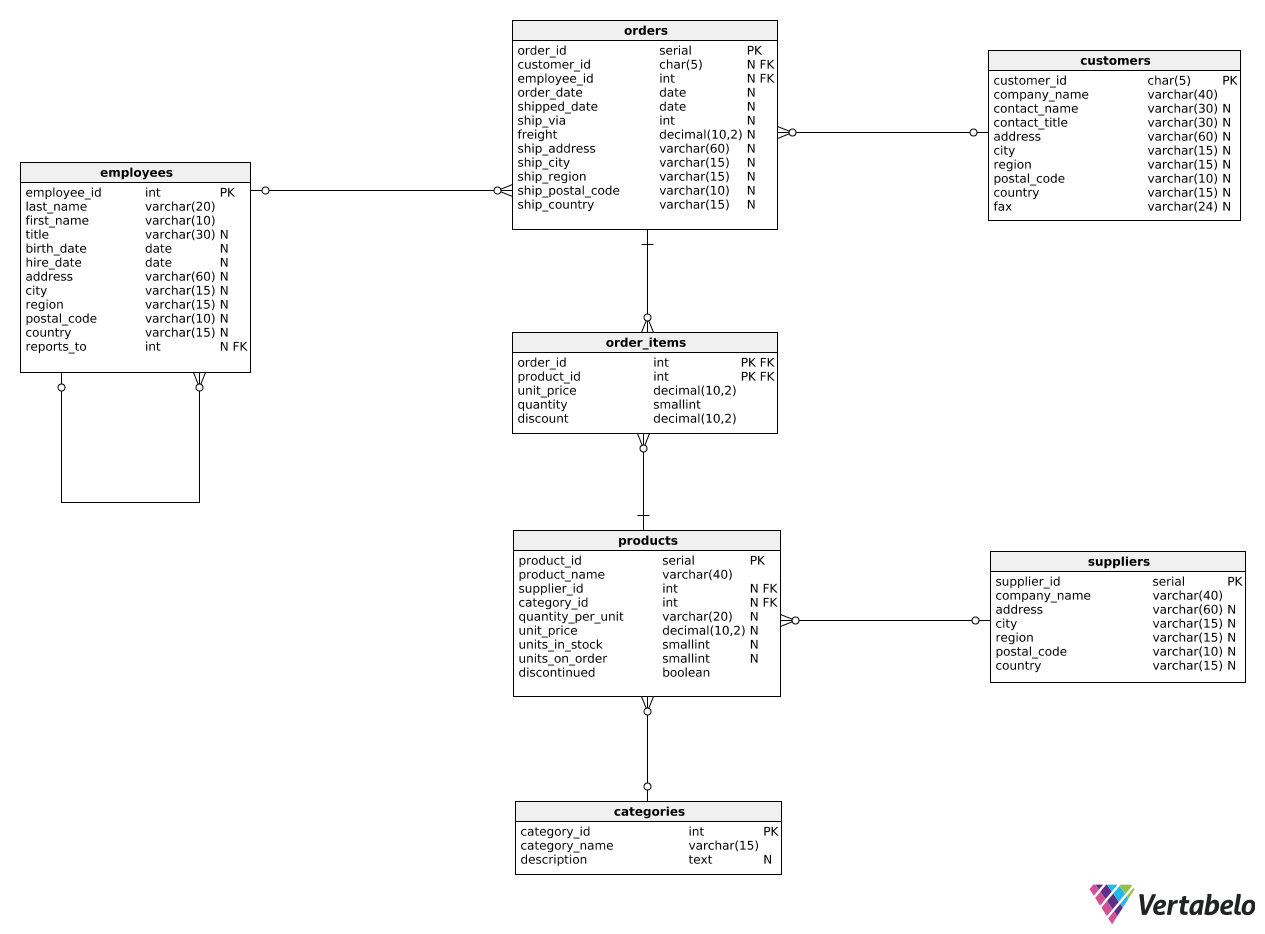Deals Of The Week - hours only!Up to 80% off on all courses and bundles.-Close
Introduction
Multiple metrics for a single object
Metrics for two groups
Ratios and percentages
Global vs. specific metrics
12. Global vs. specific metrics – explanation
Summary

## Instruction

Great! The final report type we'll talk about in this part shows what proportion of the whole each group represents.

Suppose that we want to create a report that shows information about the customers who placed orders in July 2016 and the percentage of total monthly revenue each customer generated. We can use the following code:

WITH total_sales AS (
SELECT
SUM(quantity * unit_price) AS july_sales
FROM order_items oi
JOIN orders o
ON o.order_id = oi.order_id
WHERE order_date >= '2016-07-01' AND order_date < '2016-08-01'
)
SELECT
c.customer_id,
SUM(quantity * unit_price) AS revenue,
ROUND(SUM(quantity * unit_price) / total_sales.july_sales::decimal * 100, 2) AS revenue_percentage
FROM total_sales,
customers c
JOIN orders o
ON c.customer_id = o.customer_id
JOIN order_items oi
ON oi.order_id = o.order_id
WHERE order_date >= '2016-07-01' AND order_date < '2016-08-01'
GROUP BY c.customer_id, total_sales.july_sales;


In the CTE, we simply calculate the total monthly revenue from July 2016. In the outer query, we show each customer_id alongside that customer's revenue in July 2016. But note what happens in the last column: We divide the customer's revenue (from the previous column) by the july_sales value from the CTE. This gives us the revenue percentage generated by a given customer.

Note that we had to add the july_sales column to the GROUP BY clause because it wasn't used with any aggregate function.

Naturally, the outer query only makes sense when it has the same WHERE clause as the inner query. Also, note that we join the total_sales and the customers tables in the following way:

FROM total_sales, customers c

This ensures that all rows (here, the only row) from the total_sales CTE are combined with all rows from the customers table. As a result, the july_sales value is available in all rows of the total_sales-customers combination.

## Exercise

We want to see each employee alongside the number of orders they processed in 2017 and the percentage of all orders from 2017 that they generated. Show the following columns:

1. employee_id.
2. first_name.
3. last_name.
4. order_count – the number of orders processed by that employee in 2017.
5. order_count_percentage – the percentage of orders from 2017 processed by that employee.

Round the value of the last column to two decimal places.

### Stuck? Here's a hint!

Use the following expression to calculate the percentage:

ROUND(COUNT(order_id) / total_count.all_orders::decimal * 100, 2) AS order_count_percentage


You can join tables in the following way:

FROM total_count,
employees e
JOIN orders o
ON e.employee_id = o.employee_id